Example Questions

Example Question #1 : How To Find Value With A Number Line

On a real number line, what is the midpoint of –4 and 18?

14

7

8

4

11

7

Explanation:

The integer halfway between –4 and 18 is 7. The number 7 is 11 greater than –4 and 11 less than 18. Another way to determine this would be to find the average of the two numbers: (–4 + 18)/2 = 14/2 = 7.

Example Question #2 : How To Find Value With A Number Line

On a number line, what is the distance between –6 and 7?

–13

–1

3

13

1

13

Explanation:

To find distance between two points, take the second and subtract the first.

So 7–(–6) = 7 + 6 = 13

Example Question #3 : How To Find Value With A Number Line

What is the distance on a number line betweenand?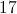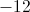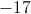Explanation:

The distance between 2 numbers on a number line is the sum of their absolute values.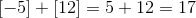Example Question #1 : How To Find Value With A Number Line

Which equation can be used to describe the following number line: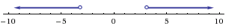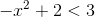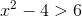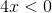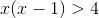Explanation:

Solve each equation for, plot on number line.

Example Question #5 : How To Find Value With A Number Line

Which number below is farthest from –3 on a number line?

–8

3/2

–1

3

0